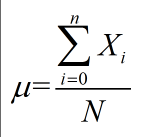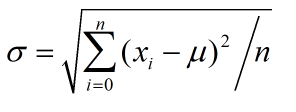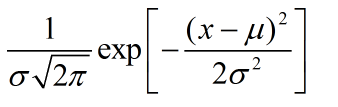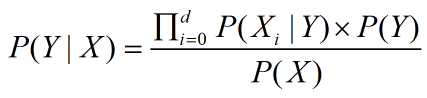# 分类算法---朴素贝叶斯算法

1年前   阅读数 128 0

## 1.数据特征类型

1.属性是离散型，类的先验概率可以通过训练各类样本出现的次数来估计

2.属性是连续型，有两种方法，将连续属性范围值划分为各个离散区间，这种方法不太好控制区间的大小，不太实用。第二种方法是，可以假设连续变量服从某种概率分布，然后使用训练数据估计分布的参数，高斯分布通常被用来表示连续属性的类条件概率分布。

高斯分布有两个参数，均值和方差.

均值计算公式（公式1）

方差计算公式（公式2）

高斯分布概率密度函数（公式3）

## 2.贝叶斯公式原型（公式4）

解释  d是每个X的属性个数,[x1,x2,x3,x4.....xd]每个属性，P(X)对于每个后验概率来说是固定不变的，所以，可以不计。

X实体属于Y分类的判断条件是根据Max[P(Yi|X)]来判断X实体属于哪个分类Y。

## 3.数据离散型贝叶斯使用例子

例子：概率模型：评分数据

物品1 物品2 物品3 物品4 物品5
Alice 1 3 3 2

P（物品5=1|Alice）=  P(Alice | 物品5=1) x P(物品5=1)  = P(物品1=1 | 物品5=1) * P(物品2=3 | 物品5=1)* P(物品3=3 | 物品5=1)*P(物品4=2 | 物品5=1) * P(物品5=1)

= 1*1/2*1/2*1/2*1/2

P（物品5=2|Alice）=  P(Alice | 物品5=2) x P(物品5=2)  = P(物品1=1 | 物品5=2) * P(物品2=3 | 物品5=2)* P(物品3=3 | 物品5=2)*P(物品4=2 | 物品5=2) * P(物品5=2)

= 0*0*0*0*0

......................................

## 4.数据连续型贝叶斯使用例子

判断车厘子和樱桃

 水果类型 鲜红值 直径 质量 未知 0.80 0.86 8

p（车厘子|unkown）=P(车厘子)*p(鲜红值|车厘子)*p(直径|车厘子)*p(质量|车厘子) / evidence

p（樱桃|unkown）=P(樱桃)*p(鲜红值|樱桃)*p(直径|樱桃)*p(质量|樱桃) / evidence

evidence=P(车厘子)*p(鲜红值|车厘子)*p(直径|车厘子)*p(质量|车厘子)+P(樱桃)*p(鲜红值|樱桃)*p(直径|樱桃)*p(质量|樱桃)；

p(鲜红值|樱桃)可通过 公式3 来计算，均值和方差，分别带入 樱桃鲜红值均值和方差，求出高斯分布概率密度，其他属性，以此类推。

spark demo

https://github.com/apache/spark/tree/master/examples/src/main/java/org/apache/spark/examples

https://github.com/apache/spark/blob/master/examples/src/main/java/org/apache/spark/examples/ml/JavaNaiveBayesExample.java

我有话说: